# 了解ES6中的箭头函数极其作用域## 执行环境

• 在函数的开头加上use strict,然后再在你的谷歌浏览中测试箭头函数吧(提示：请用谷歌浏览器v38,我当时就是被浏览器版本坑了):

```(function(){
"use strict";
// use arrow functions here
}());```

`=>`

## 新的语法

`param => expression`

```//  一个参数对应一个表达式
param => expression;// 例如 x => x+2;

// 多个参数对应一个表达式
(param [, param]) => expression; //例如 (x,y) => (x + y);

// 一个参数对应多个表示式
param => {statements;} //例如 x = > { x++; return x;};

//  多个参数对应多个表达式
([param] [, param]) => {statements} // 例如 (x,y) => { x++;y++;return x*y;};

//表达式里没有参数
() => expression; //例如var flag = (() => 2)(); flag等于2
() => {statements;} //例如 var flag = (() => {return 1;})(); flag就等于1

//传入一个表达式，返回一个对象
([param]) => ({ key: value });
//例如  var fuc = (x) => ({key:x})
var object = fuc(1);

## 箭头函数是怎么实现的

```// 当前函数
var func = function (param) {
return param.split(" ");
}
// 利用箭头函数实现
var func = param => param.split(" ");```

`func("Felipe Moura"); // returns ["Felipe", "Moura"]`

## 立即执行函数(IIFE)

`( x => x * 2 )( 3 ); // 6`

```( (x, y) => {
x = x * 2;
return x + y;
})( 3, "A" ); // "6A"```

## 相关思考

```var func = x => {
return x++;
};```

`console.log(arguments); // not defined`

`typeof``instanceof函`数也能正常检查临时函数:

```func instanceof Function; // true
typeof func; // function
func.constructor == Function; // true```

```//  有效的常规语法
(function (x, y){
x= x * 2;
return x + y;
} (3, "B") );
// 无效的箭头函数语法
( (x, y) => {
x= x * 2;
return x + y;
} ( 3, "A" ) );
// 但是可以这样写就是有效的了：
( (x,y) => {
x= x * 2;return x + y;
} )( 3,"A" );//立即执行函数```

`var instance= new func(); // TypeError: func is not a constructor`

`func.prototype; // undefined`

## 作用域

```document.body.addEventListener('click', function(evt){
console.log(this); // the HTMLBodyElement itself
});```

```function Person () {
let fullName = null;
this.getName = function () {
return fullName;
};
this.setName = function (name) {
fullName = name;
return this;
};
}
let jon = new Person();
jon.setName("Jon Doe");
console.log(jon.getName()); // "Jon Doe"

```jon.setName("Jon Doe")
.getName(); // "Jon Doe"```

```let obj = {
foo: "bar",
getIt: function () {
return this.foo;
}
};
console.log( obj.getIt() ); // "bar"```

```function Student(data){
this.name = data.name || "Jon Doe";
this.age = data.age>=0 ? data.age : -1;
this.getInfo = function () {
return this.name + ", " + this.age;
};
this.sayHi = function () {
window.setTimeout( function () {
console.log( this );
}, 100 );
}
}

let mary = new Student({
name: "Mary Lou",
age: 13
});
console.log( mary.getInfo() ); // "Mary Lou, 13"
mary.sayHi();
// window```

```function Student(data){
this.name = data.name || "Jon Doe";
this.age = data.age>=0 ? data.age : -1;
this.getInfo = function () {
return this.name + ", " + this.age;
};
this.sayHi = function () {
window.setTimeout( ()=>{
// the only difference is here
console.log( this );
}, 100 );
}
}

let mary = new Student({
name: "Mary Lou",
age: 13
});
console.log( mary.getInfo() ); // "Mary Lou, 13"
mary.sayHi();
// Object { name: "Mary Lou", age: 13, ... }```

## 有趣和有用的使用

```var arr = ['a', 'e', 'i', 'o', 'u'];
arr.forEach(vowel => {
console.log(vowel);
});```

```//在Array.map里使用箭头函数，这里我就不分析函数执行过程了。。。。

var arr = ['a', 'e', 'i', 'o', 'u'];
arr.map(vowel => {
return vowel.toUpperCase();
});
// [ "A", "E", "I", "O", "U" ]```

```var factorial = (n) => {
if(n==0) {
return 1;
}
return (n * factorial (n-1) );
}
factorial(6); // 720```

```let arr = ['a', 'e', 'i', 'o', 'u'];
arr.sort( (a, b)=> a < b? 1: -1 );```

```// EventObject, BodyElement

## 总结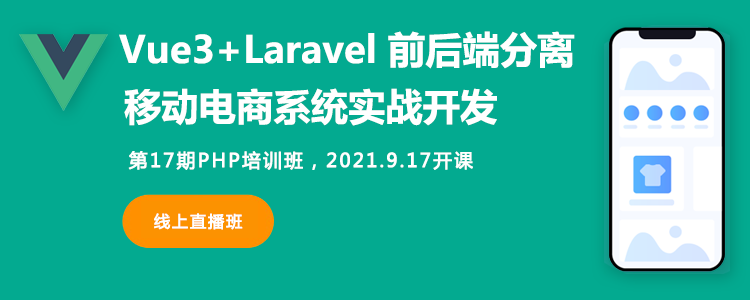#### 相关课程推荐

•## JavaScript基础精讲视频教程

《JavaScript基础精讲视频教程》本课程40多分钟带你了解JavaScript的基础，所有视频都很精简适合复习者或者有点基础的人观看，JavaScript一种直译式脚本语言，是一种动态类型、弱类型、基于原型的语言，内置支持类型。它的解释器被称为JavaScript引擎，为浏览器的一部分，广泛用于客户端的脚本语言，最早是在HTML（标准通用标记语言下的一个应用）网页上使用，用来给HTML网页增加动态功能。

JavaScript教程8173次播放

•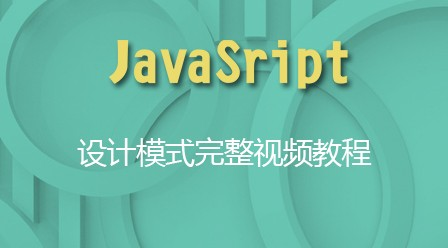## JavaScript设计模式完整视频教程

《JavaScript设计模式完整视频教程》本节视频教程是由极客学院录制，JavaScript设计模式共有两部分。第一部分给出了实现具体设计模式所需要的面向对象特性的基础知识，主要包括接口、封装和信息隐藏、继承、单体模式等内容。第二部分则专注于各种具体的设计模式及其在JavaScript语言中的应用，主要介绍了工厂模式、桥接模式、组合模式、门面模式等几种常见的模式。

JavaScript教程9174次播放

•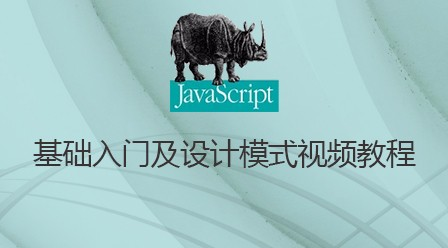## JavaScript基础入门及设计模式视频教程

《JavaScript基础入门及设计模式视频教程》该视频主要讲解JavaScript的高级设计模式，也包括JavaScript的基础入门讲解，由浅入深带大家完全掌握JavaScript设计模式。

JavaScript教程5988次播放

•## JavaScript基本语法及基本语句视频教程

《JavaScript基本语法及基本语句视频教程》本节课程是北风网录制，JavaScript一种直译式脚本语言，是一种动态类型、弱类型、基于原型的语言，内置支持类型。它的解释器被称为JavaScript引擎，为浏览器的一部分，广泛用于客户端的脚本语言，最早是在HTML（标准通用标记语言下的一个应用）网页上使用，用来给HTML网页增加动态功能。

JavaScript教程7175次播放

•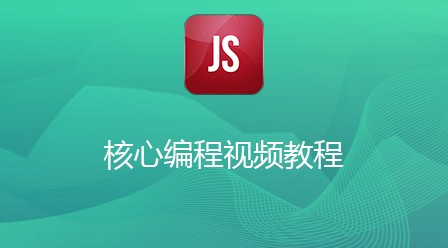## JavaScript核心编程视频教程

《JavaScript核心编程视频教程》本节课程是由北风网录制， JavaScript一种直译式脚本语言，是一种动态类型、弱类型、基于原型的语言，内置支持类型。它的解释器被称为JavaScript引擎，为浏览器的一部分，广泛用于客户端的脚本语言，最早是在HTML（标准通用标记语言下的一个应用）网页上使用，用来给HTML网页增加动态功能。

JavaScript教程7106次播放

•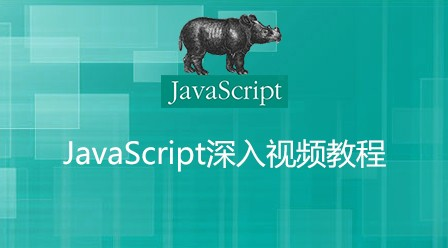## JavaScript深入视频教程

《JavaScript深入视频教程》本节课程由北风网录制，JavaScript一种直译式脚本语言，是一种动态类型、弱类型、基于原型的语言，内置支持类型。它的解释器被称为JavaScript引擎，为浏览器的一部分，广泛用于客户端的脚本语言，最早是在HTML（标准通用标记语言下的一个应用）网页上使用，用来给HTML网页增加动态功能。

JavaScript教程9488次播放

### 全部评论我要评论

• 取消发布评论发送
• 1/1